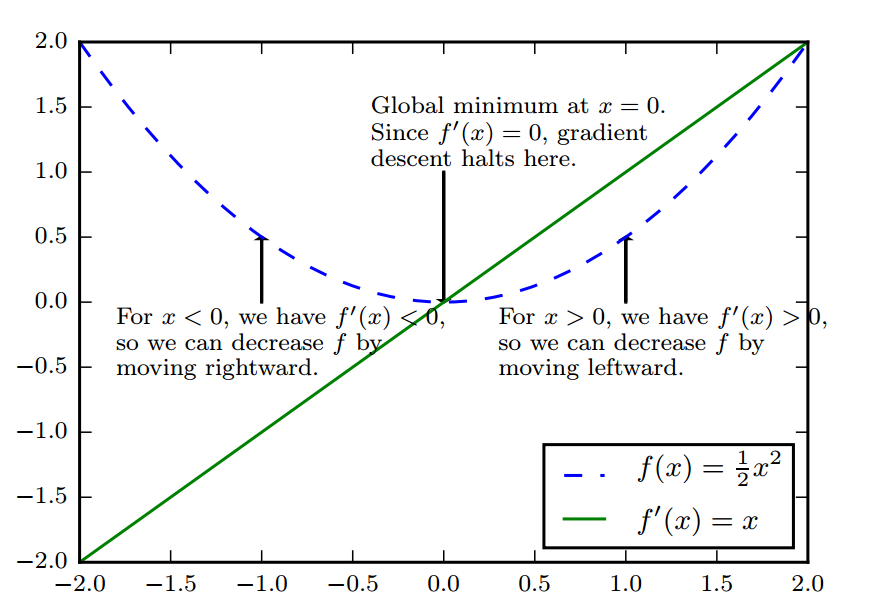# 【优化算法】基于梯度的优化算法

• 我们通常把要最大化或者最小化的函数称为目标函数(objective function) 或者准则(criterion)
• 我们通常使用一个上标$\ast$$*$ 表示最小化或最大化函数的 $x$$x$值，如 ${x}^{\ast }=\text{arg}\phantom{\rule{thickmathspace}{0ex}}minf\left(x\right)$$x^* = \text{arg} \; \min f(x)$${f}^{\prime }\left(x\right)=0$$f'(x) = 0$ 时，导数无法提供往哪个方向移动的信息，这样的点被称为临界点（critical point） 或者驻点（stationary point）。一个临界点，可能是一个 局部极小点(local minimum)， 也可能是一个 局部极大点(local maximum), 还有一类是既不极大也不极小，而是一边极大一边极小的鞍点(saddle point).

• 一种是直接设置为一个小常数。 – 这是普通青年的做法。
• 一种是通过计算，选择使得方向导数消失的步长。 – 这是解析青年的做法。
• 一种是根据几个 $\epsilon$$\varepsilon$ 是试探计算 $f\left(x-\epsilon {\mathrm{\nabla }}_{x}f\left(x\right)$$f(x - \varepsilon \nabla_x f(x)$, 并选择其中能产生最小目标值函数值得 $\epsilon$$\varepsilon$。 – 这是线搜索青年的做法。